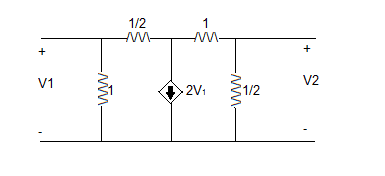# Y and z 2-port parameters

I am solving for the Y and Z parameters for the circuit below:For Y, I used the following equations by applying current sources at the terminals
va is the node at the 2v1 dependent source
(va-v1)/0.5 + (va-v2)/1 = -2v1
I1 = v1/1 + (v1-va)/0.5
I2 = v2/0.5 + (v2-va)/1
I got Y = [3 -2/3; 0 8/3]

For Z, I used KVL by applying voltage sources at the terminals
v1 = Ia - Ib
0 = Ib - Ia + 0.5Ib
0 = Ic + 0.5(Ic + Id)
v2 = 0.5(Ic + Id)
where Ia = I1, Ib = 2v1, Ic = -2v1, and Id = I2
I got Z = [1/3 1/6; 0 1/3]

I am not sure what I am doing incorrectly here. Y should be equal to Z-1, but I am not getting that. I'm not sure if my Y parameters are wrong, Z parameters, or both. :\

Last edited:

The Electrician
Gold Member
I am solving for the Y and Z parameters for the circuit below:For Y, I used the following equations by applying current sources at the terminals
va is the node at the 2v1 dependent source
(va-v1)/0.5 + (va-v2)/1 = -2v1
I1 = v1/1 + (v1-va)/0.5
I2 = v2/0.5 + (v2-va)/1
I got Y = [3 -2/3; 0 8/3]

For Z, I used KVL by applying voltage sources at the terminals
v1 = Ia - Ib
0 = Ib - Ia + 0.5Ib
0 = Ic + 0.5(Ic + Id)
v2 = 0.5(Ic + Id)
where Ia = I1, Ib = 2v1, Ic = -2v1, and Id = I2
I got Z = [1/3 1/6; 0 1/3]

I am not sure what I am doing incorrectly here. Y should be equal to Z-1, but I am not getting that. I'm not sure if my Y parameters are wrong, Z parameters, or both. :\

I think the major problem is where you have:

Ib = 2v1, Ic = -2v1

Replace those two items with Ib - Ic = 2v1

and see if you don't get better results.

when using Ib - Ic = 2v1

I got
v1 = Ia - Ib
= Ia- Ic - 2v1
= Ia + (1/3)Id - 2v1
====> v1/I1 = 1/3 and v1/I2 = 1/9
plugging in values to find the remaining parameters i got v2/I2 = 1/2 and v2/I1 = 0
so for Z parameters i got Z = [1/3 1/9; 0 1/2]

The inverse of this is [3 -2/3; 0 2] so there's still something off :\

The Electrician
Gold Member
You can't assume the voltage across the dependent source is zero; you have:

0 = Ib - Ia + 0.5Ib
0 = Ic + 0.5(Ic + Id)

You need to make those:

0 = -va + Ib - Ia + 0.5Ib
0 = va + Ic + 0.5(Ic + Id)

I'm not working out the complete solution using your method, so I may not see all the errors right away. I'm just pointing out what I see with a quick glance; you need to try to find errors also.

You might need to treat the middle two meshes together as a supermesh.

You can't assume the voltage across the dependent source is zero; you have:

0 = Ib - Ia + 0.5Ib
0 = Ic + 0.5(Ic + Id)

You need to make those:

0 = -va + Ib - Ia + 0.5Ib
0 = va + Ic + 0.5(Ic + Id)

I'm not working out the complete solution using your method, so I may not see all the errors right away. I'm just pointing out what I see with a quick glance; you need to try to find errors also.

You might need to treat the middle two meshes together as a supermesh.

are my Y parameters correct? just wondering because i don't want to be trying to use them to check if my Z parameters are correct if they aren't right in the first place..

The Electrician
Gold Member
Yes, they are correct.The Electrician
Gold Member
Have you had any luck deriving the Z parameters?

Have you had any luck deriving the Z parameters?

yep, thanks. i just had a few algebraic errors that threw me off.